Author: Oscar Cronquist Article last updated on January 13, 2023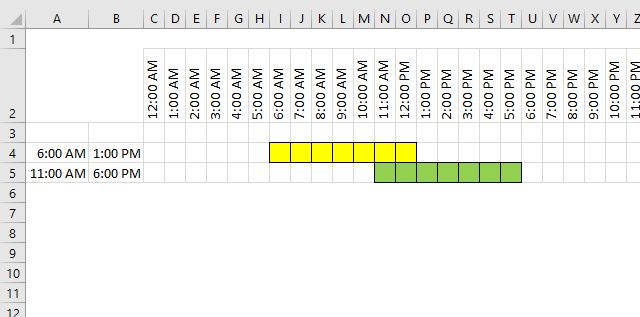I found an old post that I think is interesting to write about today. Think of two overlapping ranges, it may be dates, times, or any numerical range, the formula demonstrated here works with everything.

This picture shows two time ranges, 06:00-13:00 (yellow) and 11:00-18:00 (green).

It is obvious that there are two overlapping hours but how do we calculate how much they overlap in Excel?

The MEDIAN function comes to the rescue, but first, let me explain the function. It returns a value that separates the higher half of a data set from the lower half. Example, MEDIAN(1,2,3) returns 2. 1 is the lower half and 3 is the higher half.

MEDIAN(1,2,3,4,5,6) returns 3.5 because there are two values (3, 4) separating the higher half (5,6) from the lower half (1,2). The average of these two values is 3.5.

## 1. Calculate overlapping hours

We have 4 times here to remember, the start and end of time range 1 and 2.Let see what happens if we use the MEDIAN function with the start and end value of time range 1 and only the start value of time range 2.

MEDIAN("6:00 AM", "01:00 PM", "11:00 AM") returns 11:00 AM.

And then the end of time range 2.

MEDIAN("6:00 AM", "01:00 PM", "06:00 PM") returns 01:00 PM

01:00 PM - 11:00 AM is 02:00. Two hours are overlapping.

The formula becomes

MEDIAN(Start1,End1,End2) - MEDIAN(Start1,End1,Start2)

and returns 02:00.

## 2. Calculate total cost based on different rates per hour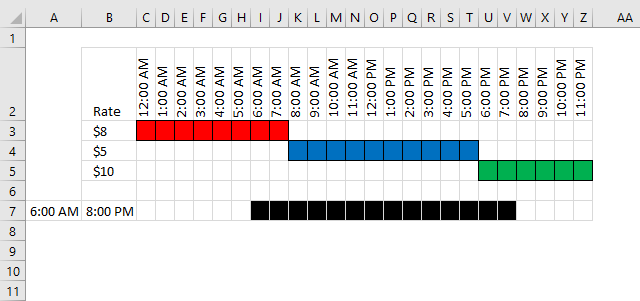The rate is 8 between 12:00 AM and 08:00 AM, 08:00 AM - 6:00 PM the rate is 5 and 6:00 PM to 12:00 AM the rate is 10.

How do we calculate total cost if the time range is 06:00 AM - 8:00 PM?

Count overlapping hours for the first range 00:00-08:00 and multiply with rate 8.

=HOUR(MEDIAN("06:00 AM", "8:00 PM", "08:00 AM") - MEDIAN("06:00 PM","8:00 PM", "12:00 AM"))*8

returns 16.

Count overlapping hours for the second range 08:00-18:00 and multiply with rate 5.

=HOUR(MEDIAN("06:00 AM", "8:00 PM", "06:00 PM") - MEDIAN("06:00 AM","8:00 PM", "08:00 AM"))*5

returns 50

Count overlapping hours for the second range 18:00-24:00 and multiply with rate 10.

=HOUR(MEDIAN("06:00 AM", "8:00 PM", "12:00 AM") - MEDIAN("06:00 AM","8:00 PM", "06:00 PM"))*10

returns 20.

Combining all formulas gives

=HOUR(MEDIAN("06:00 AM", "8:00 PM", "08:00 AM") - MEDIAN("06:00 AM","8:00 PM", "12:00 AM"))*8+HOUR(MEDIAN("06:00 AM", "8:00 PM", "06:00 AM") - MEDIAN("06:00 AM","8:00 PM", "08:00 AM"))*5+HOUR(MEDIAN("06:00 AM", "8:00 PM", "12:00 AM") - MEDIAN("06:00 AM","8:00 PM", "06:00 AM"))*10

returns 86.

### 2.1 I have a question for you

It would be great to build an array formula to shrink the formula above, like this one:

=SUM((MEDIAN(B2:B4,C2:C4,B7+{1;1;1})-MEDIAN(B2:B4,C2:C4,A7+{1;1;1}))*D2:D4)

But it won't work, you can't use the MEDIAN function to do that. Do you know a workaround? See next section.

## 3. Calculate total cost based on different rates per hour (smaller formula)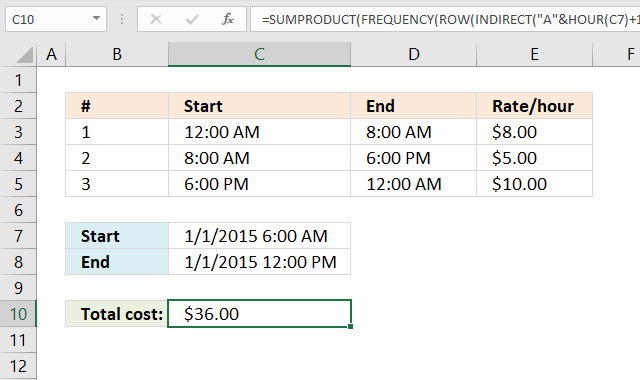The image above demonstrates a formula that calculates the total cost based on rates per hour. Check out Alex Grobermans formula in the comments section below.

=SUMPRODUCT(FREQUENCY(ROW(INDIRECT("A"&HOUR(C7)+1&":A"&HOUR(C8))), HOUR(D3:D4)), E3:E5)

The formula above won't work if you start and end spans over multiple days, see next section below.

### Explaining calculation in cell C10

#### Step 1 - Calculate hour

The HOUR function returns an integer representing the hour of an Excel time value.

HOUR(C7)

becomes

HOUR(42005.25)

and returns 6.

#### Step 2 - Create cell reference

The INDIRECT function creates a cell reference based on text values.

INDIRECT("A"&HOUR(C7)+1&":A"&HOUR(C8))

becomes

INDIRECT("A"&6+1&":A"&12)

becomes

INDIRECT("A"&7&":A"&12)

becomes

INDIRECT("A7:A12")

and returns A7:A12.

#### Step 3 - Create row numbers

The ROW function returns row numbers from a cell reference.

ROW(INDIRECT("A"&HOUR(C7)+1&":A"&HOUR(C8)))

becomes

ROW(A7:A12)

and returns {7; 8; 9; 10; 11; 12}.

#### Step 4 - Calculate frequency based on time intervals

The FREQUENCY function calculates how often values occur within a range of values and returns a vertical array of numbers. It returns an array that is one more item larger than the bins_array.

FREQUENCY(data_array, bins_array)

FREQUENCY(ROW(INDIRECT("A"&HOUR(C7)+1&":A"&HOUR(C8))), HOUR(D3:D4))

becomes

FREQUENCY({7; 8; 9; 10; 11; 12}, {8;18})

and returns {2; 4; 0}.

#### Step 5 - Multiply with rates and return a total

The SUMPRODUCT function calculates the product of corresponding values and then returns the sum of each multiplication.

SUMPRODUCT(array1, [array2], ...)

SUMPRODUCT(FREQUENCY(ROW(INDIRECT("A"&HOUR(C7)+1&":A"&HOUR(C8))), HOUR(D3:D4)), E3:E5)

becomes

SUMPRODUCT({2; 4; 0}, E3:E5)

becomes

SUMPRODUCT({2; 4; 0}, {8;5;10})

and returns 36.

## 4. Calculate total cost based on different rates per hour across days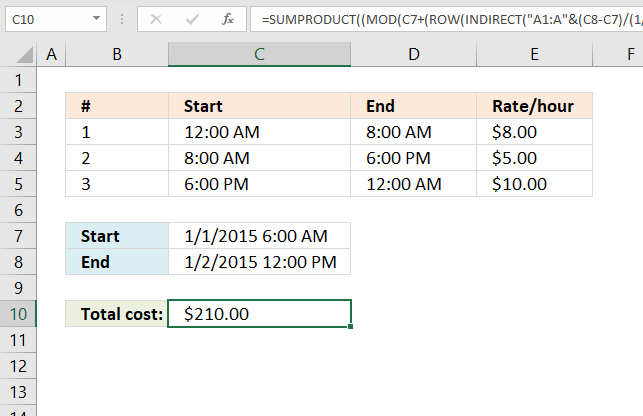Alex Groberman contributed with an interesting formula, check out that comment below. I modified that formula and came up with this in order to get it working with a range that spans over multiple days.

Array formula in cell C10:

=SUMPRODUCT((MOD(C7+(ROW(INDIRECT("A1:A"&(C8-C7)/(1/24)))-1)/24, 1)<TRANSPOSE(D3:D5))*(MOD(C7+(ROW(INDIRECT("A1:A"&(C8-C7)/(1/24)))-1)/24, 1)>=TRANSPOSE(C3:C5))*TRANSPOSE(E3:E5))

The formula above works with all Excel versions, the formula below is smaller, however, it works only in Excel 365:

=LET(x, MOD(C7+(SEQUENCE(, (C8-C7)/(1/24))-1)/24, 1), SUMPRODUCT((x<D3:D5)*(x>=C3:C5)*E3:E5))

### Explaining calculation in cell C10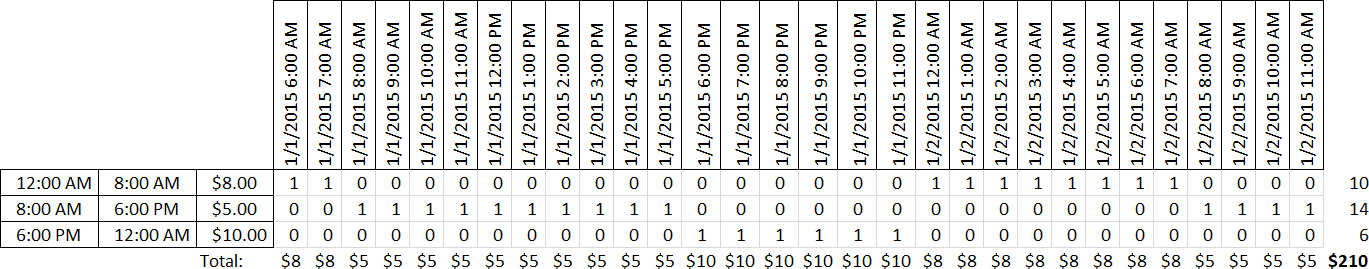Press with left mouse button on the image above to see a larger version. The image shows the time range from start (cell C7) 1/1/2015 6:00 AM to end (cell C8) 1/1/2015 12:00 PM.